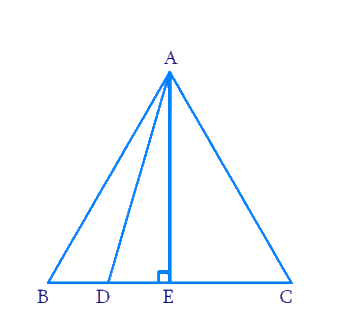# Ex.6.5 Q15 Triangles Solution - NCERT Maths Class 10

## Question

In an equilateral triangle $$ABC$$, $$D$$ is a point on side $$BC$$ such that $$BD$$ $$=$$\begin{align}\frac{1}{3}BC\end{align}

Prove that $$9A{{D}^{2}}=7AB{}^{2}$$ .

DiagramVideo Solution
Triangles
Ex 6.5 | Question 15

## Text Solution

Reasoning:

In a right triangle, the square of the hypotenuse is equal to the sum of the squares of the other two sides.

Steps:

\begin{align} \text{In}\,\,\Delta A B C ; & \,\, A B=B C=C A \\ & \text{and}\,\,BD=\frac{1}{3}BC \\ & \text{Draw} \,AE\bot BC \\ & BE=CE=\frac{1}{2}BC \end{align}

[ $$\because$$ In an equilateral triangle perpendicular drawn from vertex to opposite side bisects the side]

Now In $$\Delta ADE$$

\begin{align} A{{D}^{2}} & =AE{}^{2}+D{{E}^{2}} \\ & [ \text { Pythagoras } \text{ theorem } ] \end{align}

\begin{align}=(\frac{\sqrt{3}}{2}BC)+{{(BE-BD)}^{2}}\end{align}

[ $$\because$$ $$AE$$ is the height of an equilateral triangle which is equal to \begin{align}\frac{\sqrt{3}}{2}\end{align} side]

\begin{align}A{D^2} &= \frac{3}{4}B{C^2} + {\left[ {\frac{{BC}}{2} - \frac{{BC}}{3}} \right]^2}\\A{D^2} &= \frac{3}{4}B{C^2} + {\left( {\frac{{BC}}{6}} \right)^2}\\ A{D^2} &= \frac{3}{4}B{C^2} + \frac{{B{C^2}}}{{36}}\\A{D^2} &= \frac{{27B{C^2} + B{C^2}}}{{36}}\\36A{D^2} &= 28B{C^2}\\9A{D^2} &= 7B{C^2}\\9A{D^2} &= 7A{B^2} \\& \left[ {AB \!= \!BC\! = \!CA} \right]\end{align}

Learn from the best math teachers and top your exams

• Live one on one classroom and doubt clearing
• Practice worksheets in and after class for conceptual clarity
• Personalized curriculum to keep up with school